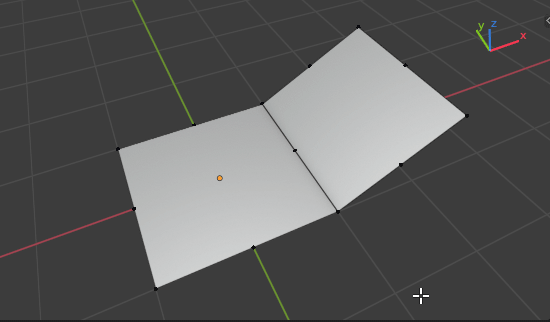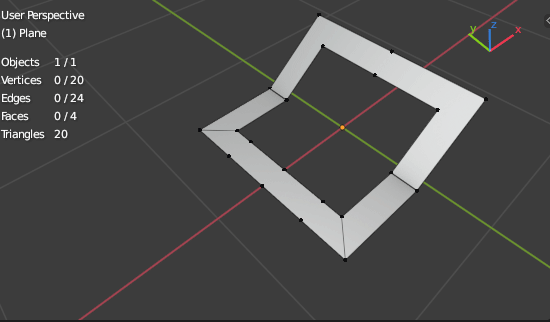# [Solved] Select vertices with only 2 edges connected on flat edge

Example:

If I use Select Similar - Amount of Adjacent Faces or Amount of Connected Edges

it select verts that makes shape

Is it possible after Select Similar deselect vertices that have angle not equal 180 degree?
Or maybe somehow select all vertices that have only 2 edges and 180 degree between?

I don’t think there’s a built-in way to do that. You can do it from a script or the Python console by selecting all vertices that only link to two edges and whose edges have an (absolute) dot product close to 1.0.
On the console, something like this (untested):

``````import bmesh
bm = bmesh.from_edit_mesh(C.object.data)
for v in bm.verts:
v.select = selectColinear(v)
bmesh.update_edit_mesh(C.object.data)
``````

Edit: updated to fix the errors discussed below.

1 Like

thanks.
Slightly modified but I think something is missing here

``````import bpy, bmesh
import numpy as np

me = bpy.context.object.data
bm = bmesh.from_edit_mesh(me)
bm.verts.foreach_set('select', [selectColinear(vertex) for vertex in bm.verts])
bmesh.update_edit_mesh(me)
``````

AttributeError: ‘BMVertSeq’ object has no attribute ‘foreach_set’

Yeah, that’s the “untested” part heh.
In the absence of a `foreach_set()` in a collection, just manually iterate over each vertex and set their `select` attribute:

``````(...)
for vertex in bm.verts:
vertex.select = selectColinear(vertex)
bmesh.update_edit_mesh(me)``````
1 Like

If you only need to use it on that shape, I thought of a couple of methods:

Method 1: press A to select all vertices, then C to go Circle Select mode and just deselect the corners. Not that big of a deal.

Method 2: select all corners then press Ctrl + I to invert the selection (selecting every vertex that isn’t a corner).

1 Like

No, its for checking bad topology in complex mesh, you just one click found them and dissolve.

As I can found lambda needs its own context to hold its value
so to not get this warning it needs to add something more yet
`AttributeError: Vector subtraction: (Vector - BMVert) invalid type for this operation`
but anyway thanks for helping!

In that case, would the existing `bpy.ops.mesh.dissolve_limited()` operator in vertex mode do what you want? https://docs.blender.org/api/blender2.8/bpy.ops.mesh.html?highlight=dissolve_limited#bpy.ops.mesh.dissolve_limited

It has no issue cleaning up your example mesh above for example.

1 Like

That’s a typo, I forgot to access `.co` in this expression:

``````.dot(v.co - v.link_edges.other_vert(v))
``````

It should be:

``````.dot(v.co - v.link_edges.other_vert(v).co)
``````

That’s a built-in operation called Limited Dissolve. No need for a script x)

1 Like

Learn new about blender every day!
Thanks for helping!

1 Like

Hi! Thanks for the code snippet! I turned it into an operator (there is more work can be done but that’s what it looks like for now):

``````import bmesh
import bpy
from bpy.props import BoolProperty, FloatProperty

class SelectCollinearVertices(bpy.types.Operator):
"""Select collinear vertices with 2 connected edges"""
bl_idname = "mesh.select_collinear_vertices"
bl_label = "Select Collinear Vertices"
bl_options = {'REGISTER', 'UNDO'}

factor: FloatProperty(name="Factor", default=0.9, min=0.0, max=1.0)
extend_selection: BoolProperty(name="Extend Selection", default=False)

@classmethod

def poll(cls, context):
ob = context.active_object
return all([bool(ob), ob.type == "MESH", ob.mode == "EDIT"])

def select_collinear(self, v):

if len(le) == 2:
return (abs((v.co - le.other_vert(v).co).dot(v.co - le.other_vert(v).co)) > self.factor)

return False

def execute(self, context):
me = context.object.data
bm = bmesh.from_edit_mesh(me)
bm.select_mode = {'VERT'}

for v in bm.verts:
if not (self.extend_selection and v.select):
v.select = self.select_collinear(v)

bm.select_flush_mode()
bmesh.update_edit_mesh(me)
return {'FINISHED'}

def register():
bpy.utils.register_class(SelectCollinearVertices)

def unregister():
bpy.utils.unregister_class(SelectCollinearVertices)

if __name__ == "__main__":
register()
``````3 Likes

Wow, yes, thank you!

I did not want bothering people but Limit Dissolve does not do what I want exactly
Example

as you can see Limit Dissolve also dissolving some supported loops and edges.

After some testing I changed the behavior a bit so there is an ability to use actual angle values for selecting vertices.

Operator can be found under Shift+G (select similar) menu while in vertex edit mode.``````from math import pi

import bmesh
import bpy
from bpy.props import BoolProperty, FloatProperty
from bpy.types import VIEW3D_MT_edit_mesh_select_similar

class SelectVerticesByAngle(bpy.types.Operator):
"""Select vertices with 2 connected edges by angle between them"""
bl_idname = "mesh.select_vertices_by_angle"
bl_label = "Select Vertices By Angle"
bl_options = {'REGISTER', 'UNDO'}

def update_min_angle(self, context):
if self.min_angle > self.max_angle:
self.max_angle = self.min_angle

def update_max_angle(self, context):
if self.max_angle < self.min_angle:
self.min_angle = self.max_angle

min_angle: FloatProperty(name="Min Angle", subtype='ANGLE',
default=pi * 0.75, min=0.0, max=pi,
update=update_min_angle)
max_angle: FloatProperty(name="Max Angle", subtype='ANGLE',
default=pi, min=0.0, max=pi,
update=update_max_angle)
extend: BoolProperty(name="Extend", default=False)

@classmethod
def poll(cls, context):
ob = context.active_object
return all([bool(ob), ob.type == "MESH", ob.mode == "EDIT"])

def select_by_angle(self, v):

if len(le) == 2:
vec1 = v.co - le.other_vert(v).co
vec2 = v.co - le.other_vert(v).co
angle = abs((vec1).angle(vec2))

return (angle >= self.min_angle and angle <= self.max_angle)

return False

def execute(self, context):
me = context.object.data
bm = bmesh.from_edit_mesh(me)
bm.select_mode = {'VERT'}

for v in bm.verts:
if not (self.extend and v.select):
v.select = self.select_by_angle(v)

bm.select_flush_mode()
bmesh.update_edit_mesh(me)

return {'FINISHED'}

def check(self, context):
return True

def draw_select_vertices_by_angle(self, context):
mesh_select_mode = context.scene.tool_settings.mesh_select_mode

if tuple(mesh_select_mode) == (True, False, False):
layout = self.layout
layout.separator()
layout.operator(SelectVerticesByAngle.bl_idname,
text="Vertices By Angle")

def register():
bpy.utils.register_class(SelectVerticesByAngle)
VIEW3D_MT_edit_mesh_select_similar.append(draw_select_vertices_by_angle)

def unregister():
bpy.utils.unregister_class(SelectVerticesByAngle)
VIEW3D_MT_edit_mesh_select_similar.remove(draw_select_vertices_by_angle)

if __name__ == "__main__":
register()``````
2 Likes